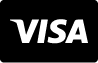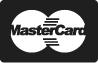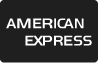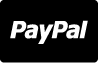Get 10 FREE Images when you get started on our 1 Month-Free Trial.

# 免版税图像 - Math chalkboard vector illustration. Physics solving equation blackboard. Sketch with geometrical class problem solution or algebra formula. Higher mathematics Intelligence or complex calculation mess

Math chalkboard vector illustration. Physics solving equation blackboard. Sketch with geometrical class problem solution or algebra formula. Higher mathematics Intelligence or complex calculation mess

5500 x 3667 px | 18.33 " x 12.22 " | 300dpi | JPG

1 866 655 3733